# Inequalities On A Number Line Worksheet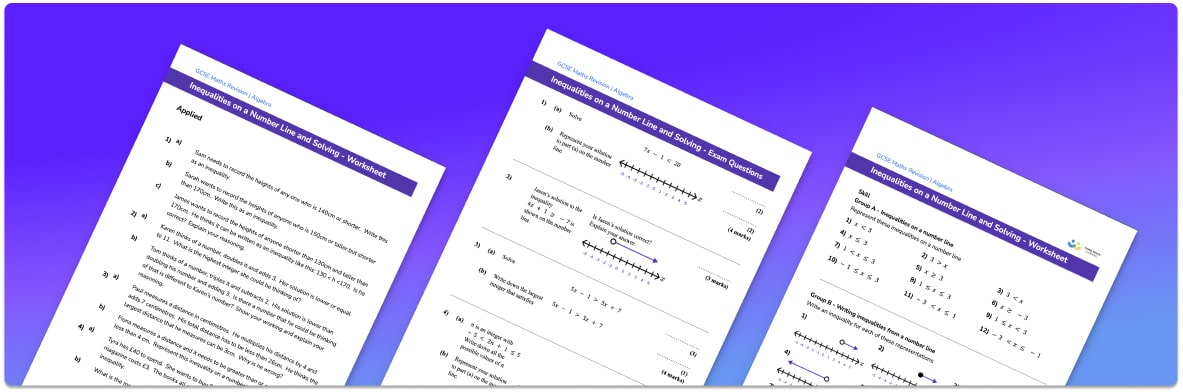Help your students prepare for their Maths GCSE with this free inequalities on a number line worksheet of 30+ questions and answers

• Section 1 of the inequalities on a number line printable worksheet contains 20+ skills-based inequalities on a number line  questions, in 3 groups to support differentiation
• Section 2 contains 3 applied inequalities on a number line  questions with a mix of worded problems and deeper problem solving questions
• Section 3 contains 3 foundation and higher level GCSE inequalities on a number line exam questions
• Answers and a mark scheme for all inequalities on a number line  questions are provided
• Questions follow variation theory with plenty of opportunities for students to work independently at their own level
• All questions created by fully qualified expert secondary maths teachers
• Suitable for GCSE maths revision for AQA, OCR and Edexcel exam boards
• To receive this resource and regular emails with more free resources, blog posts and other Third Space updates, enter your email address and click below.

• This field is for validation purposes and should be left unchanged.

You can unsubscribe at any time (each email we send will contain an easy way to unsubscribe). To find out more about how we use your data, see our privacy policy.

### Inequalities on a number line at a glance

Inequalities demonstrate relationships between two expressions that are not equal to each other. There are four types of inequality symbols: greater than >, less than <, greater than or equal to ≥ and less than or equal to ≤. For example, x>4 means x is greater than 4 , so x could be 5, 6, 7, … etc.

Inequalities can be represented on a number line. This gives us a visual representation of the possible values a variable can take. When showing inequalities on a number line, we use two different types of circle. We use an open circle if the inequality does not include an ‘equals to’ and a closed circle if it does. We then use an arrow from the circle or a line between two circles to indicate the possible values the variable can take.

Solving algebraic inequalities such as linear inequalities and quadratic inequalities is very similar to solving algebraic equations, but instead of using an equals sign we use an inequality symbol. As with linear equations, we can have one step inequalities, two step inequalities and unknowns on each side of the inequality. The solution to an inequality represents a range of values rather than one number and the solution set can be represented on a number line.

Given an inequality, we can also write down the possible values a variable can take. We are usually interested in the relevant integer values (whole numbers) it can take. For example, if 3≤x<8 then the possible integer values of x are 3, 4, 5, 6, and 7.

Looking forward, students can then progress to additional algebra worksheets, for example a solving equations worksheet or simultaneous equations worksheet.For more teaching and learning support on Algebra our GCSE maths lessons provide step by step support for all GCSE maths concepts.

## Related worksheets

Solving Quadratic Inequalities Worksheet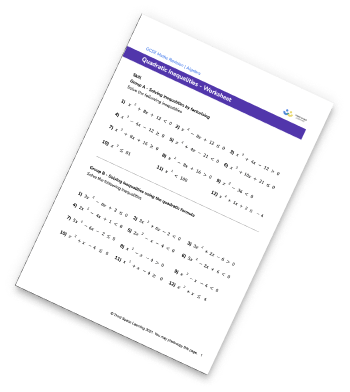Composite and Inverse Functions Worksheet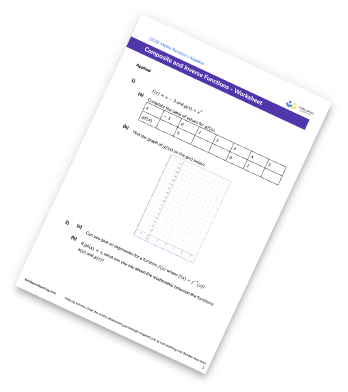Quadratic Sequences and Quadratic Nth Term Worksheet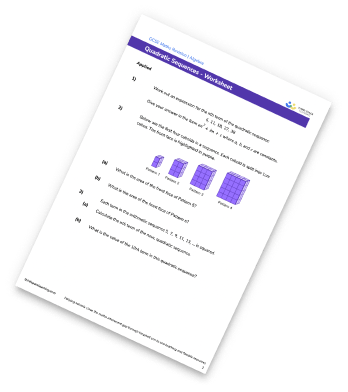Brackets with Indices Worksheet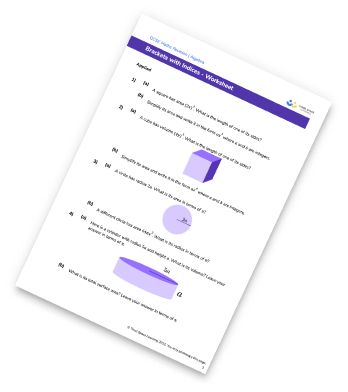## Do you have KS4 students who need more focused attention to succeed at GCSE?There will be students in your class who require individual attention to help them succeed in their maths GCSEs. In a class of 30, it’s not always easy to provide.

Help your students feel confident with exam-style questions and the strategies they’ll need to answer them correctly with our dedicated GCSE maths revision programme.

Lessons are selected to provide support where each student needs it most, and specially-trained GCSE maths tutors adapt the pitch and pace of each lesson. This ensures a personalised revision programme that raises grades and boosts confidence.

Find out more